JEE  >  Test: Slope Of A Line

# Test: Slope Of A Line

Test Description

## 10 Questions MCQ Test Mathematics (Maths) Class 11 | Test: Slope Of A Line

Test: Slope Of A Line for JEE 2023 is part of Mathematics (Maths) Class 11 preparation. The Test: Slope Of A Line questions and answers have been prepared according to the JEE exam syllabus.The Test: Slope Of A Line MCQs are made for JEE 2023 Exam. Find important definitions, questions, notes, meanings, examples, exercises, MCQs and online tests for Test: Slope Of A Line below.
Solutions of Test: Slope Of A Line questions in English are available as part of our Mathematics (Maths) Class 11 for JEE & Test: Slope Of A Line solutions in Hindi for Mathematics (Maths) Class 11 course. Download more important topics, notes, lectures and mock test series for JEE Exam by signing up for free. Attempt Test: Slope Of A Line | 10 questions in 10 minutes | Mock test for JEE preparation | Free important questions MCQ to study Mathematics (Maths) Class 11 for JEE Exam | Download free PDF with solutions
 1 Crore+ students have signed up on EduRev. Have you?
Test: Slope Of A Line - Question 1

### The value of y, for the line passing through (3, y) and (2, 7) is parallel to the line passing through (-1 , 4) and (0, 6) is:

Detailed Solution for Test: Slope Of A Line - Question 1

As A(3,y) and B(2,7) is parallel to C(-1,4) and D(0,6)
∴ Their slopes are equal
so, (y-7)/(3-1) = (4-6)/(-1-0)
y-7 = 2
y = 9

Test: Slope Of A Line - Question 2

### The tangent of the angle which the part of the line above the X-axis makes with the positive direction of the X-axis is:

Detailed Solution for Test: Slope Of A Line - Question 2

The gradient or slope of a line (not parallel to the axis of y) is the trigonometrical tangent of the angle which the line makes with the positive direction of the x-axis. Thus, if a line makes an angle θ with the positive direction of the x-axis, then its slope will be tan θ.

Test: Slope Of A Line - Question 3

### Two lines are said to be parallel when the difference of their slopes is:

Detailed Solution for Test: Slope Of A Line - Question 3

Parallel lines and their slopes are easy. Since slope is a measure of the angle of a line from the horizontal, and since parallel lines must have the same angle, then parallel lines have the same slope and lines with the same slope are parallel.

Test: Slope Of A Line - Question 4

Slope of a line which cuts intercepts of equal lengths on the axes is:

Detailed Solution for Test: Slope Of A Line - Question 4

The equation of line which cuts intercepts of equal lengths on the axes is: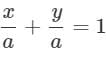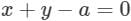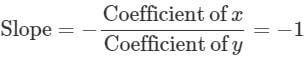Test: Slope Of A Line - Question 5

The points A and B have coordinates (3, 2) and (1, 4) respectively. So, the slope of any line perpendicular to AB is:

Detailed Solution for Test: Slope Of A Line - Question 5

If the lines are perpendicular to each other then their slopes are in the form m1.m2 = -1.(since product of slopes of two perpendicular lines is -1) Therefore , m = 1.

Test: Slope Of A Line - Question 6

Slope of a line is not defined, when q =

Detailed Solution for Test: Slope Of A Line - Question 6

Since tan θ is not defined when θ = 90°, therefore, the slope of a vertical line is not defined. i.e., slope of y-axis is m = tan 90° = ∞ i.e., not defined.

Test: Slope Of A Line - Question 7

If the slope of the line passing through the points (2, 5) and (x, 1) is 2, then x = ______.

Detailed Solution for Test: Slope Of A Line - Question 7

Slope = Change in y coordinates÷change in x coodinates
= (5-1)÷(2-x) =2
5-1 = 4-2x
0 = 2x
x=0

Test: Slope Of A Line - Question 8

If the slope of line m = tan 0°. Therefore, the line is _____ to the X-axis.

Detailed Solution for Test: Slope Of A Line - Question 8

Slope of x-axis is m = tan 0° = 0.
Since the inclination of every line parallel to x-axis is 0°, so its slope (m) = tan 0° = 0. Therefore, the slope of every horizontal line is 0.

Test: Slope Of A Line - Question 9

If A (-2, 1), B (2, 3) and C (-2, -4) are three points, find the angle between the straight lines AB and BC.

Detailed Solution for Test: Slope Of A Line - Question 9

Let the slope of the line AB and AC are m1 and m2  respectively.

Then,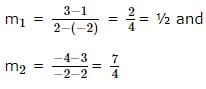Let θ be the angle between AB and BC. Then,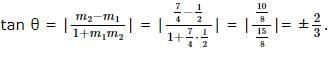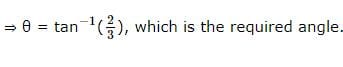Test: Slope Of A Line - Question 10

Let A(2, 12) and B(6,4) be two points. The slope of a line perpendicular to the line AB is:

Detailed Solution for Test: Slope Of A Line - Question 10

Slope of the points A and B is -2 and the lines are perpendicular then

m1×m2 = -1.

∴ the slope is 1/2

## Mathematics (Maths) Class 11

156 videos|176 docs|132 tests
 Use Code STAYHOME200 and get INR 200 additional OFF Use Coupon Code
Information about Test: Slope Of A Line Page
In this test you can find the Exam questions for Test: Slope Of A Line solved & explained in the simplest way possible. Besides giving Questions and answers for Test: Slope Of A Line, EduRev gives you an ample number of Online tests for practice

## Mathematics (Maths) Class 11

156 videos|176 docs|132 tests